## RS Aggarwal Class 10 Solutions Chapter 3 Linear equations in two variables MCQS

These Solutions are part of RS Aggarwal Solutions Class 10. Here we have given RS Aggarwal Solutions Class 10 Chapter 3 Linear equations in two variables MCQS.

### RS Aggarwal Solutions Class 10 Chapter 3

Choose the correct answer in each of the following questions.
Question 1.
Solution:
(c)Question 2.
Solution:
(c)Question 3.
Solution:
(a)Question 4.
Solution:
(d)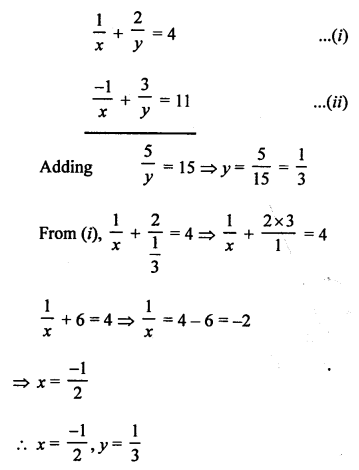Question 5.
Solution:
(a)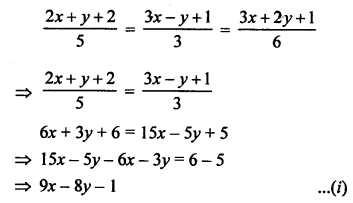Question 6.
Solution:
(b)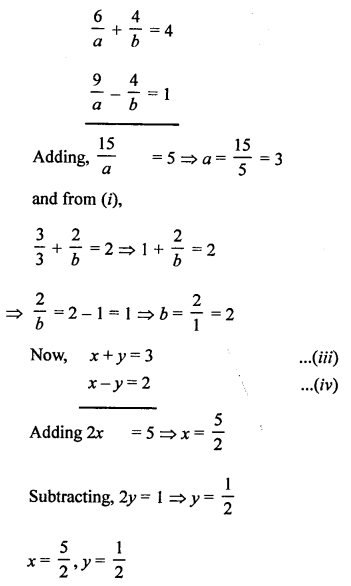Question 7.
Solution:
(c) 4x + 6y = 3xy, 8x + 9y = 5xy
Dividing each term by xy,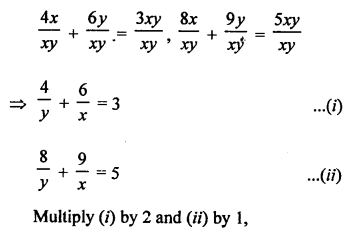Question 8.
Solution:
(a)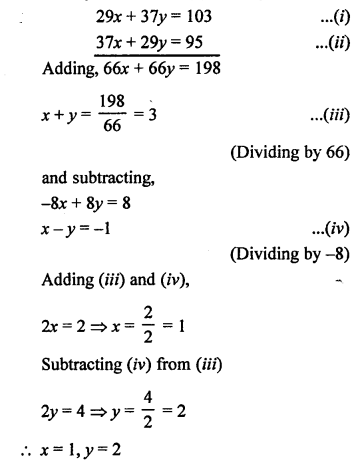Question 9.
Solution:
(c)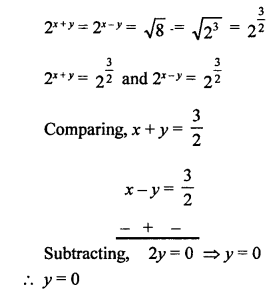Question 10.
Solution:
(b)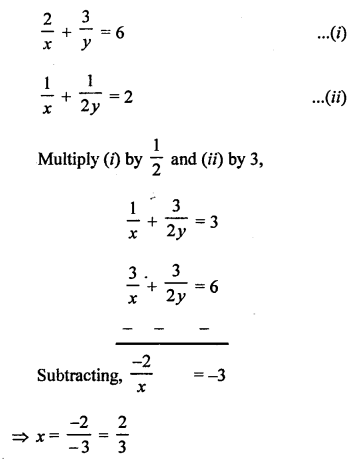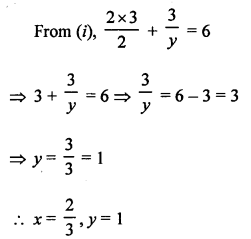Question 11.
Solution:
(d)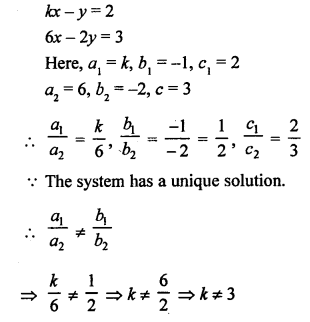Question 12.
Solution:
(b)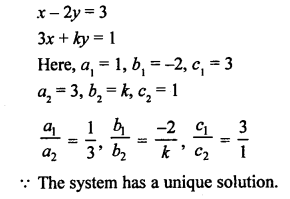Question 13.
Solution:
(a)Question 14.
Solution:
(d)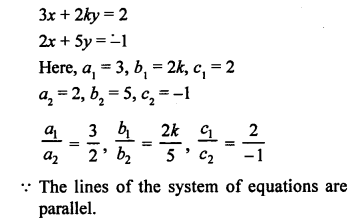Question 15.
Solution:
(d)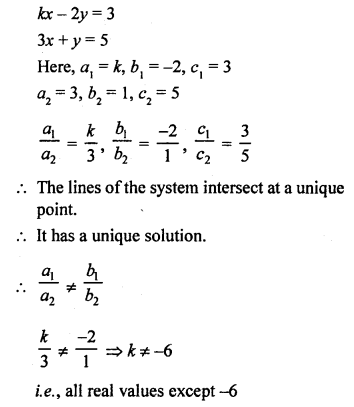Question 16.
Solution:
(d)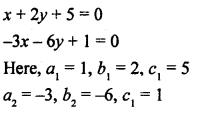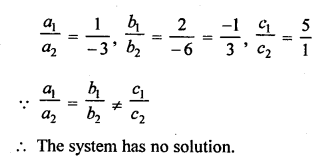Question 17.
Solution:
(d)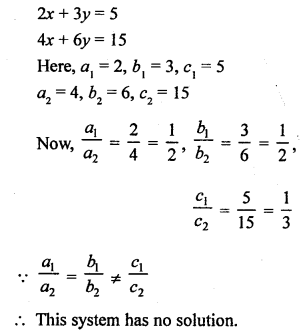Question 18.
Solution:
(d) The system of equations is consistent then their graph lines will be either intersecting or coincident.

Question 19.
Solution:
(a) The pair of lines of equation is inconsistent then the system will not have no solution i.e., their lines will be parallel.

Question 20.
Solution:
(b)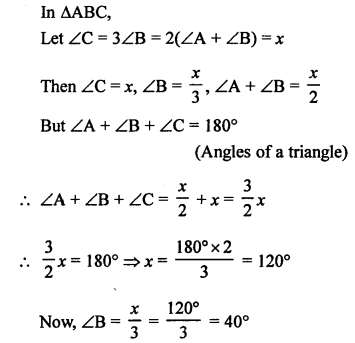Question 21.
Solution:
(b) ABCD is a cyclic quadrilateral
∠A = (x + y + 10)°, ∠B = (y + 20)°, ∠C = (x + y – 30)° and ∠D = (x + y)°
∠A + ∠C = 180°
Now, x + y + 10°+ x + y – 30° = 180°
⇒ 2x + 2y – 20 = 180°
⇒ 2x + 2y = 180° + 20° = 200°
⇒ x + y = 100° …(i)
and ∠B + ∠D = 180°
⇒ y + 20° + x + y = 180°
⇒ x + 2y = 180° – 20° = 160° …(ii)
Subtracting,
-y = -60° ⇒ y = 60°
and x + 60° = 100°
⇒ x = 100° – 60° = 40°
Now, ∠B = y + 20° = 60° + 20° = 80°

Question 22.
Solution:
(d) Let one’s digit of a two digit number = x
and ten’s digit = y
Number = x + 10y
By interchanging the digits,
One’s digit = y
and ten’s digit = x
Number = y + 10x
According to the conditions,
x + y = 15 …(i)
y + 10x = x + 10y + 9
⇒ y + 10x – x – 10y = 9
⇒ 9x – 9y = 9
⇒ x – y = 1 …(ii)
2x = 16 ⇒ x = 8
and x + y = 15
⇒ 8 + y = 15
⇒ y = 15 – 8 = 7
Number = x + 10y = 8 + 10 x 7 = 8 + 70 = 78

Question 23.
Solution:
(b) Let the numerator of a fractions = x
and denominator = y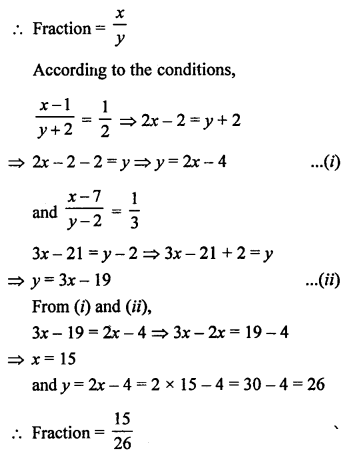Question 24.
Solution:
(d) Let present age of man = x years
and age of his son = y years
5 years hence,
Age of man = (x + 5) years
and age of son = y + 5 years
(x + 5) = 3 (y + 5)
⇒ x + 5 = 3y + 15
x = 3y + 15 – 5
x = 3y + 10 ……(i)
and 5 years earlier
Age of man = x – 5 years
and age of son = y – 5 years
x – 5 = 7 (y – 5)
x – 5 = 7y – 35
⇒ x = 7y – 35 + 5
x = 7y – 30 ……….(ii)
From (i) and (ii),
7y – 30 = 3y + 10
⇒ 7y – 3y = 10 + 30
⇒ 4y = 40
y = 10
x = 3y + 10 = 3 x 10 + 10 = 30 + 10 = 40
Present age of father = 40 years

Question 25.
Solution:
(b)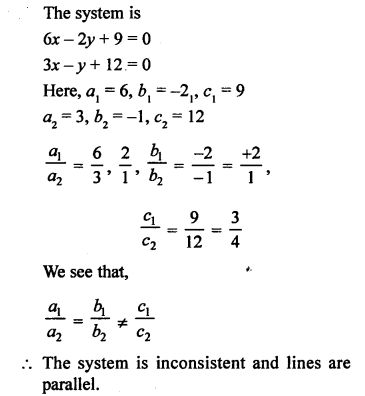Question 26.
Solution:
(c)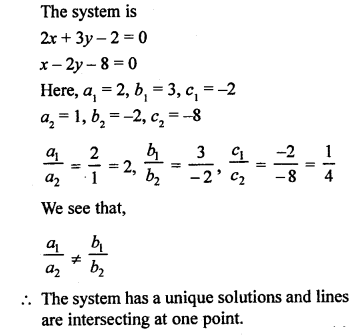Question 27.
Solution:
(a)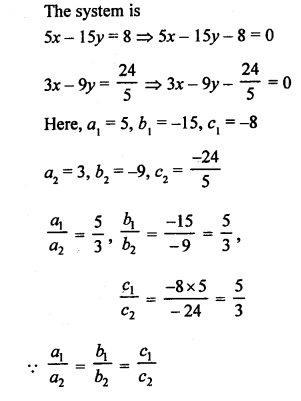The system has infinitely many solutions.
The lines are coincident.

Hope given RS Aggarwal Solutions Class 10 Chapter 3 Linear equations in two variables MCQS are helpful to complete your math homework.

If you have any doubts, please comment below. Learn Insta try to provide online math tutoring for you.